Publication date: 11/10/2021

## Goal Plot

This section provides details about the calculation of the mean shift and standard deviation standardized to specification quantities plotted in the Goal Plot. This section uses the notation defined in Notation for Goal Plots and Capability Box Plots.

The mean shift and the standard deviation standardized to the specification limits for the jth column are defined as follows:

Spec-Standardized Mean =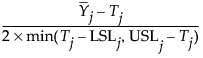Spec-Standardized Std Dev =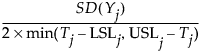Note: If either LSLj or USLj is missing, twice the distance from the target to the nonmissing specification limit is used in the denominators of the Goal Plot coordinates.

### Goal Plot Points for Processes with Missing Targets

Suppose that the process has both a lower and an upper specification limit but no target. Then the formulas given in Goal Plot are used, replacing Tj with the midpoint of the two specification limits.

Suppose that the process has only one specification limit and no target. To obtain (x,y) coordinates for a point on the Goal Plot, the capability indices of the process are used. (See Capability Indices for Normal Distributions for definitions in terms of the theoretical mean and standard deviation.) For sample observations, the following relationships hold: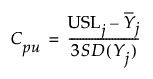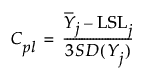If a process has two specification limits and a target at the midpoint of the limits, then the (x,y) coordinates for the point on the Goal Plot satisfy these relationships: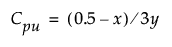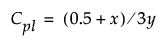To obtain coordinates when there is only one specification limit and no target, these relationships are used. To identify a unique point requires an assumption about the slope of the line from the origin on which the points fall. A slope of 0.5 is assumed for the case of an upper specification limit and of -0.5 for a lower specification limit. When capability values are equal to one and the Ppk slider for the goal plot triangle is set to 1, these slopes place the points on the goal plot triangle lines.

Consider the case of only an upper specification limit and no target. Using the assumption that the (x,y) coordinates fall on a line from the origin with slope 0.5, solving for x and y gives the following coordinates: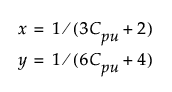Consider the case of only a lower specification limit and no target. Using the assumption that the (x,y) coordinates fall on a line from the origin with slope -0.5, solving for x and y gives the following coordinates: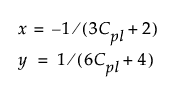Note: If either Cpu or Cpl is less than -0.6, then it is set to -0.6 in the formulas above. At the value -2/3, the denominator for x assumes the value 0. Bounding the capability values at -0.6 prevents the denominator from assuming the value 0 or switching signs.